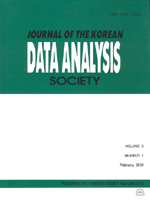상세검색
최근 검색어 전체 삭제
다국어입력
즐겨찾기0KCI등재 학술저널

# Binary Forecast of Haze over Busan

• 등재여부 : KCI등재
• 2016.02
• 31 - 38 (8 pages)

The objective of the present study is to develop the binary forecast model of haze over Busan. For forecast modeling, three kinds of data are used. The first one is the observations at Beijing and Qingdao in the global telecommunication system. The second one is observed meteorological factors at Korea Meteorological Administration and the third one is the outputs from community multi-scale air quality model. All of data are converted to daily data for easy application to daily forecast system. Haze occurrence is a dichotomous response variable. A logistic regression model is applied to generate probabilistic forecasts of haze occurrence. Predictors are selected based on correlation analysis and multicollinearity check. A threshold is required to improve the forecast quality of binary forecast. A binary forecast model consists of a probabilistic forecast model and the optimal threshold which maximizes the CSI. As results, the optimal threshold is 0.4 and AC is 86.6%, 56.9% for POD, 29.6% for FAR and 45.9% for CSI.

1. Introduction

2. Data and Methodology

3. Binary forecast model for haze over Busan

4. Concluding Remarks

References